top of page
Search
• Nagesh Singh Chauhan

# Classifying Heart Disease Using K-Nearest Neighbors

### I have written this post for the developers and assumes no background in statistics or mathematics. The focus is mainly on how the k-NN algorithm works and how to use it for predictive modeling problems.Classification of objects is an important area of research and application in a variety of fields. In the presence of full knowledge of the underlying probabilities, Bayes decision theory gives optimal error rates. In those cases where this information is not present, many algorithms make use of distance or similarity among samples as a means of classification.

The article has been divided into 2 parts. In the first part, we’ll talk all about the K-NN machine learning algorithm and in the second part, we will implement K-NN in real life and classify Heart disease patients.

Table of content

1. What is a K-NN algorithm?

2. How does the K-NN algorithm work?

3. When to choose K-NN?

4. How to choose the optimal value of K?

5. What is Curse of dimensionality?

6. Building K-NN classifier using python sci-kit learn.

7. How to improve the performance of your classifier?

# What is a K-NN Algorithm?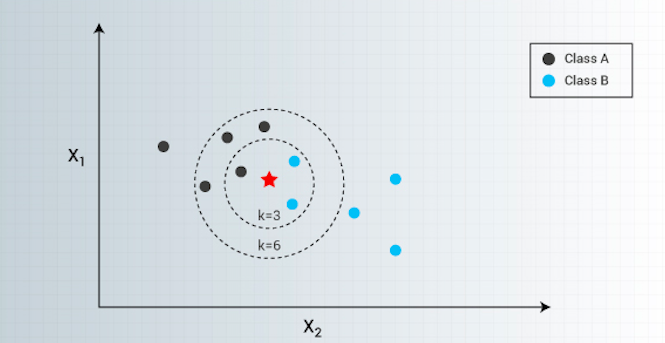K-NN or K-Nearest Neighbors is one of the most famous classification algorithms as of now in the industry simply because of its simplicity and accuracy.

K-NN is a simple algorithm that stores all available cases and classifies new cases based on a similarity measure (e.g., distance functions). KNN has been used in statistical estimation and pattern recognition already at the beginning of the 1970s as a non-parametric technique.

The algorithm assumes that similar things exist in close proximity. In other words, entities which are similar exist together.

# How the K-NN algorithm works?

In K-NN, K is the number of nearest neighbors. The number of neighbors is the core deciding factor. K is generally an odd number if the number of classes is 2. When K=1, then the algorithm is known as the nearest neighbor algorithm. This is the simplest case.

In the below figure, suppose yellow colored “?” let's say P is the point, for which label needs to predict. First, you find the one closest point to P and then the label of the nearest point assigned to P.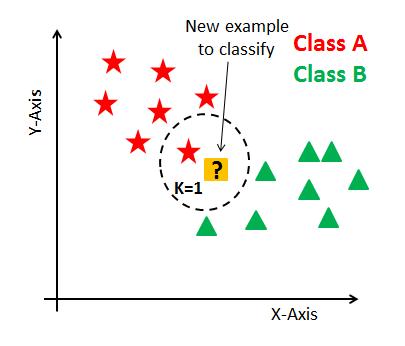Second, you find the k closest point to P and then classify points by majority vote of its K neighbors. Each object votes for their class and the class with the most votes is taken as the prediction. For finding closest similar points, we find the distance between points using distance measures such as Euclidean distance, Hamming distance, Manhattan distance, and Minkowski distance. The algorithm has the following basic steps:

1. Calculate distance

2. Find closest neighbors

3. Vote for labelsThree most commonly used distance measures used to calculate the distance between point P and its nearest neighbors are represented as :Euclidean distance: It is the most commonly used distance measure also called simply distance. The usage of a Euclidean distance measure is highly recommended when the data is dense or continuous. Euclidean distance is the best proximity measure. The Euclidean distance between two points is the length of the path connecting them. The Pythagorean theorem gives this distance between two points.

Below figure shows how to calculate Euclidean distance between two points in a 2-dimensional plane.# When to use K-NN algorithm?

KNN can be used for both classification and regression predictive problems. However, it is more widely used in classification problems in the industry. To evaluate any technique we generally look at 3 important aspects:

1. Ease to interpret the output 2. Calculation time of the algorithm 3. Predictive Power

Let us compare KNN with different models: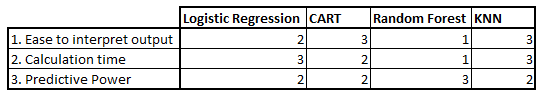Credits: Analytics Vidhya

As you can see K-NN surpasses Logistic Regression, CART and Random Forest in terms of the aspects which we are considering.

# How to choose the optimal value of K?

The number of neighbors(K) in K-NN is a hyperparameter that you need to choose at the time of building your model. You can think of K as a controlling variable for the prediction model.

Now, choosing the optimal value for K is best done by first inspecting the data. In general, a large K value is more precise as it reduces the overall noise but there is no guarantee. Cross-validation is another way to retrospectively determine a good K value by using an independent dataset to validate the K value. Historically, the optimal K for most datasets has been between 3–10. That produces much better results than 1NN(when K=1).

Generally, an odd number is chosen if the number of classes is even. You can also check by generating the model on different values of K and check their performance.

# Curse of Dimensionality

K-NN performs better with a lower number of features than a large number of features. You can say that when the number of features increases than it requires more data. Increase in dimension also leads to the problem of overfitting. To avoid overfitting, the needed data will need to grow exponentially as you increase the number of dimensions. This problem of higher dimension is known as the Curse of Dimensionality.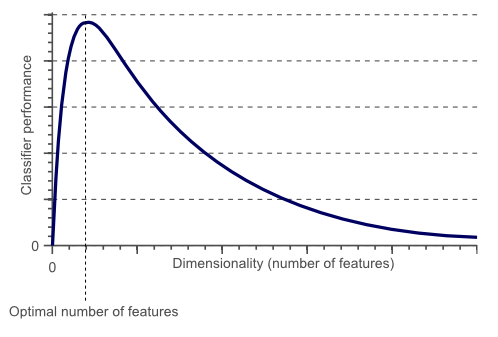From the above graphical representation, it is clearly visible that the performance of your model decreases with an increase in the number of features(dimensions).

To deal with the problem of the curse of dimensionality, you need to perform principal component analysis(PCA) before applying any machine learning algorithm, or you can also use feature selection approach. Research has shown that in large dimension Euclidean distance is not useful anymore. Therefore, you can prefer other measures such as cosine similarity, which get decidedly less affected by high dimension.

The KNN algorithm can compete with the most accurate models because it makes highly accurate predictions. Therefore, you can use the KNN algorithm for applications that require high accuracy but that do not require a human-readable model. — source: IBM

Steps to compute K-NN algorithm:

1. Determine parameter K = number of nearest neighbors. 2. Calculate the distance between the query-instance and all the training samples. 3. Sort the distance and determine nearest neighbors based on the K-th minimum distance. 4. Gather the category of the nearest neighbors 5. Use a simple majority of the category of nearest neighbors as the prediction value of the query.

In the next section, we are going to solve a real world scenario using K-NN algorithm.

# Building Heart disease classifier using K-NN algorithmThe most crucial task in the healthcare field is disease diagnosis. If a disease is diagnosed early, many lives can be saved. Machine learning classification techniques can significantly benefit the medical field by providing an accurate and quick diagnosis of diseases. Hence, save time for both doctors and patients. As heart disease is the number one killer in the world today, it becomes one of the most difficult diseases to diagnose.

In this section, we are going to build a K-NN classifier which will predict the presence of heart disease in a patient or not.

This database contains 76 attributes, but all published experiments refer to using a subset of 14 of them. In particular, the Cleveland database is the only one that has been used by ML researchers to this date. The “goal” field refers to the presence of heart disease in the patient. It is integer valued from 0 (no presence) to 4.

Dataset contains following features:

age — age in years sex — (1 = male; 0 = female) cp — chest pain type trestbps — resting blood pressure (in mm Hg on admission to the hospital) chol — serum cholestoral in mg/dl fbs — (fasting blood sugar > 120 mg/dl) (1 = true; 0 = false) restecg — resting electrocardiographic results thalach — maximum heart rate achieved exang — exercise induced angina (1 = yes; 0 = no) oldpeak — ST depression induced by exercise relative to rest slope — the slope of the peak exercise ST segment ca — number of major vessels (0–3) colored by flourosopy thal — 3 = normal; 6 = fixed defect; 7 = reversable defect target — have disease or not (1=yes, 0=no)

Lets begin…

```import numpy as np
import matplotlib.pyplot as plt
import pandas as pd
import seaborn as sns
from sklearn.model_selection import train_test_split
from sklearn.preprocessing import StandardScaler
from sklearn.neighbors import KNeighborsClassifier
from sklearn.metrics import confusion_matrix
from sklearn import metrics```

`data = pd.read_csv('/Users/nageshsinghchauhan/Downloads/ML/KNN_art/heart.csv')`Original dataset

Let us explore our dataset and count the number of patients who have heart disease:

```data.target.value_counts()1    165
0    138
Name: target, dtype: int64```

So out of all the patients 165 patients actually have heart disease. Now also visualize.

```sns.countplot(x="target", data=data, palette="bwr")
plt.show()```Count of the number of patients having heart disease(target =1)

Now let's classify target variable between male and female and visualize the result.

```sns.countplot(x='sex', data=data, palette="mako_r")
plt.xlabel("Sex (0 = female, 1= male)")
plt.show()```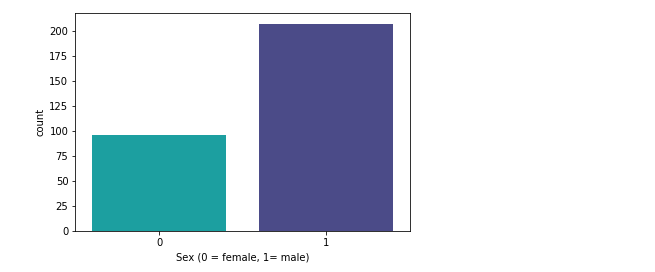Count of the male and female having heart disease

So from the above figure, it is evident that in our dataset, 207 males and 96 females are there.

Let us also see the relation between “Maximum Heart Rate” and “Age”.

```plt.scatter(x=data.age[data.target==1], y=data.thalach[(data.target==1)], c="green")
plt.scatter(x=data.age[data.target==0], y=data.thalach[(data.target==0)], c = 'black')
plt.legend(["Disease", "Not Disease"])
plt.xlabel("Age")
plt.ylabel("Maximum Heart Rate")
plt.show()```Scatter plot between Age and Maximum heart rate

So from the above, the maximum heart rate occurs in between age 50–60 years.

Ok, now let's label our dataset with X(matrix of independent variables) and y(vector of the dependent variable).

```X = data.iloc[:,:-1].values
y = data.iloc[:,13].values```

Next, we split 75% of the data to the training set while 25% of the data to test set using below code.

`X_train, X_test, y_train, y_test =  train_test_split(X,y,test_size = 0.25, random_state= 0)`

Now, Our dataset contains features which are highly varying in magnitudes, units, and range. But since most of the machine learning algorithms use Euclidean distance between two data points in their computations, this is a problem. To suppress this effect, we need to bring all features to the same level of magnitudes. This can be achieved by a method called feature scaling.

So our next step is to normalize the data which can be done using StandardScaler() from sci-kit learn.

```sc_X = StandardScaler()
X_train = sc_X.fit_transform(X_train)
X_test = sc_X.transform(X_test)```

Our next step is to K-NN model and train it with the training data. Here n_neighbors is the value of factor K.

```classifier = KNeighborsClassifier(n_neighbors = 5, metric = 'minkowski', p = 2)
classifier = classifier.fit(X_train,y_train)```

So the most important point to note here is to choose the optimal value of K and for that, we will start with K=5.

Now, since your K-NN model is ready with K=5. Let's train our test data and check its accuracy.

```y_pred = classifier.predict(X_test)
#check accuracy
accuracy = metrics.accuracy_score(y_test, y_pred)
print('Accuracy: {:.2f}'.format(accuracy))```
Accuracy: 0.82

For K=6

```classifier = KNeighborsClassifier(n_neighbors = 6, metric = 'minkowski', p = 2)
classifier = classifier.fit(X_train,y_train)
#prediction
y_pred = classifier.predict(X_test)
#check accuracy
accuracy = metrics.accuracy_score(y_test, y_pred)
print('Accuracy: {:.2f}'.format(accuracy))```
Accuracy: 0.86

For K=7

```classifier = KNeighborsClassifier(n_neighbors = 7, metric = 'minkowski', p = 2)
classifier = classifier.fit(X_train,y_train)
#prediction
y_pred = classifier.predict(X_test)
#check accuracy
accuracy = metrics.accuracy_score(y_test, y_pred)
print('Accuracy: {:.2f}'.format(accuracy))```
Accuracy: 0.87

For K=8

```classifier = KNeighborsClassifier(n_neighbors = 8, metric = 'minkowski', p = 2)
classifier = classifier.fit(X_train,y_train)
#prediction
y_pred = classifier.predict(X_test)
#check accuracy
accuracy = metrics.accuracy_score(y_test, y_pred)
print('Accuracy: {:.2f}'.format(accuracy))```
Accuracy: 0.87

For K=9

```classifier = KNeighborsClassifier(n_neighbors = 9, metric = 'minkowski', p = 2)
classifier = classifier.fit(X_train,y_train)
#prediction
y_pred = classifier.predict(X_test)
#check accuracy
accuracy = metrics.accuracy_score(y_test, y_pred)
print('Accuracy: {:.2f}'.format(accuracy))```
Accuracy: 0.86

So as we can see that Accuracy is maximum that is 87% when K=7.

Let's also check the confusion matrix and see how many records were predicted correctly.

```#confusion matrix
from sklearn.metrics import confusion_matrix
cm = confusion_matrix(y_test, y_pred)```
array([[26, 7], [ 3, 40]])

In the output, 26 and 40 are correct predictions, and 7 and 3 are incorrect predictions.

# How to improve the performance of your classifier?

Well, there are many ways in which the KNN algorithm can be improved.

• Changing the distance measure for different applications may help improve the accuracy of the algorithm. (i.e. Hamming distance for text classification).

• Dimensionality reduction techniques like PCA should be executed prior to applying K-NN

• Rescaling your data makes the distance measure more meaningful. For instance, given 2 features height and weight, an observation such as z=[220,60]z=[220,60] will clearly skew the distance metric in favor of height. One way of fixing this is by column-wise subtracting the mean and dividing by the standard deviation.

• Approximate Nearest Neighbor techniques such as using k-d trees to store the training data can be performed to decrease testing time.

## Conclusion

Congratulations, you have successfully built a heart disease classifier using K-NN which is capable of classifying heart patient with optimal accuracy.

In this article, we have learned the K-NN, it’s working, the curse of dimensionality, model building and evaluation on heart disease dataset using Python Scikit-learn package.